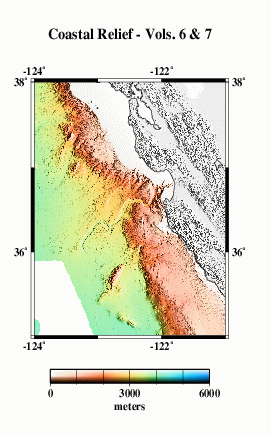# Multibeam Swath Bathymetry - Southern California

## Data### Grid Information:

• West = -124
• East = -121
• South = 35
• North = 38
• Grid Nodes: nx = 3601, ny = 3601
• Depth: Max = 4204 m, Min = -1789 m

### GMT command line arguments:

• -R-124/-121/35/38
• -I3c

## Profile Comparisons

Here we compare profiles from the NGDC Coastal Relief Model (A) with estimated bathymetry (B), and satellite-derived gravity anomalies (C). Because of the resolution limit of the altimeter data used in the bathymetry prediction, the estimated anomalies do not resolve the very short-wavelength seafloor features evident in the Coastal Relief Model (A).

## Grid Analysis

We compare the difference between altimetry-estimated bathymetry (2 min. grid) and the Coastal Relief Model (3 sec. grid) two ways, because the two are on grids of different spacing and thus may capture different length scales of bathymetric roughness.

For the lower-resolution analysis (H and I), Coastal Relief Model data were averaged into corresponding 2-arc-minute blocks, and estimated bathymetry was subtracted.

For the higher-resolution analysis (J and K), bilinear interpolation was used to obtain estimated bathymetry values at each point on the Coastal Relief Model grid, then the estimated bathymetry was subtracted.

In both cases, we find the mean difference is ~ 3 m (the Coastal Relief Model data are systematically deeper); the difference is about .2% of the depth. An error like this is expected because of the uncertainties in whether or not data sets used in calibration have been corrected for the variable speed of sound in seawater, errors translating uncorrected sound speeds between nominal fathoms and nominal meters, etc.

The standard deviations about the mean are 116 m for the 2 arcmin averages and 106 m for the model points. This result is opposite of what we expect- the standard deviations should be greater for the high resolution differences. This might be due in part to the high number of constrained points in the estimated bathymetry.

Eventually, we want to do a detailed cross-spectral analysis to determine how much of the actual Coastal Relief Model is captured in the altimeter gravity field. Here, we present a very simplified model. We take the Coastal Relief Model (A) and simply calculate a model gravity field, assuming that all the topography has the same density and is entirely uncompensated. This model (M) does not look much like the observed gravity (L), because, in fact, most of the topographic relief in this region is compensated. The satellite gravity reflects deeper density structures, while the Coastal Relief Model maps the compensated seafloor topography.

The radially-averaged power spectra of the two (N) show the simple gravity model has more power at most wavelengths. At wavelengths greater than ~100 km or so, this is due to the effects of isostatic compensation of the topography that is not taken into account in the model. At shorter wavelengths the model also has more power than the altimetric gravity. Some of this is due to smoothing in the altimeter data necessary to remove noise. We don't know if the simple model is realistic at these wavelengths. We need high quality ship gravity data to compare.